## Example Questions

### Example Question #1221 : Act Science

A researcher is investigating new solar technology. The researcher looks at combinations of solar panels and solar rechargeable batteries that were provided from research labs A, B, C and D. The combinations of solar panels and solar rechargeable batteries are measured for their efficiency, the amount of time it takes to fully charge a battery, and the life of each fully charged battery used to power a home. The experiments were conducted using a UV light. The batteries recharge when the solar panels are exposed to UV light and are depleted of charge when being used for power. The solar panels will not recharge the batteries on cloudy days.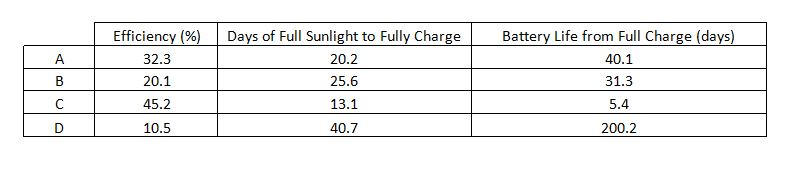If the researcher could combine the solar panel from one of the labs with the batteries from another lab, then which combination would give the highest efficiency and longest battery life? Assume that the solar panels and batteries are all compatible with no adverse effects.

Solar panels from D and rechargable batteries from B

Solar panels from D and rechargable batteries from C

Cannot be determined

Solar panels from C and rechargable batteries from D

Solar panels from C and rechargable batteries from B

Solar panels from C and rechargable batteries from D

Explanation:

The solar panel from lab C has the highest efficiency at 45.2%. Since there are no compatibility issues it can be assumed that battery life and solar panel efficiency measurements will remain the same. The battery from lab D has the longest battery life (200.2 days). The correct answer is to use the solar panels from lab C and the batteries from lab D.

### Example Question #1231 : Act Science

A researcher is investigating new solar technology. The researcher looks at combinations of solar panels and solar rechargeable batteries that were provided from research labs A, B, C and D. The combinations of solar panels and solar rechargeable batteries are measured for their efficiency, the amount of time it takes to fully charge a battery, and the life of each fully charged battery used to power a home. The experiments were conducted using a UV light. The batteries recharge when the solar panels are exposed to UV light and are depleted of charge when being used for power. The solar panels will not recharge the batteries on cloudy days.If the equipment from research lab D was under the same UV light as in the experiment, what is the expected percent efficiency?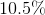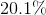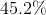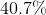Cannot be determinedExplanation:

Since the same UV light is being used as in the laboratory it can be expected that the results from an experiment would be the same. This means that the percent efficiency for setup D will be the same: 10.5 percent efficiency.

### Example Question #1232 : Act Science

Engineers are evaluating four potential technologies. These technologies are to be used as power plants that are considered "clean" energy. The estimated energy output of these plants were calculated and the resources needed to run these were also listed.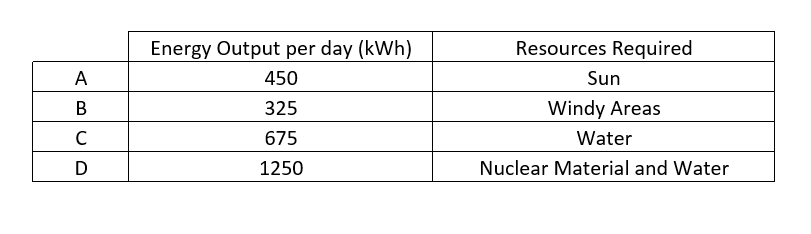If all other resources are readily available, which technology should be pursued in costal communities?

A

B

D

C

D

Explanation:

Costal communities have access to water and all other technologies are available. This allows for the community to use the technology with the highest energy output. This energy output is 1250kWh and is from option D, which is the correct answer.

### Example Question #1233 : Act Science

A company developed new prototype car and wanted to test the new car. The car's acceleration time from 0mph to 60mph and stopping time from 60mph to 0mph were measured. An obstacle course involving a lot of turns was also constructed to determine how well the car can handle turning. The main concern of the company is the safety of the car and therefore all of the tests were performed on dry concrete, concrete after simulated rain, concrete that was covered with snow and in sand.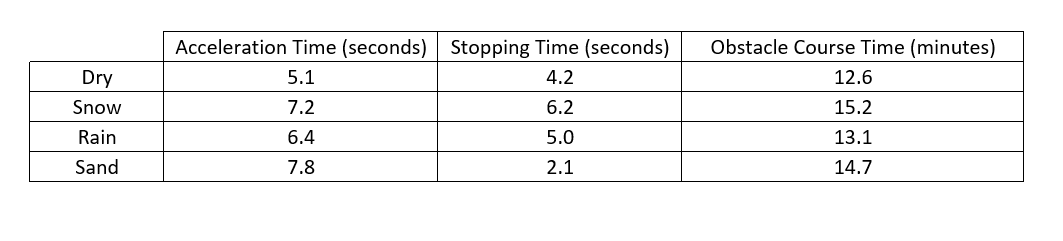What is true about the acceleration time and stopping time?

As the acceleration time increases so does the stopping time

As acceleration time decreases the stopping time increases

The stopping time is always shorter than the acceleration time in the same conditions

As the acceleration time increases the stopping time decreases

The stopping time is always shorter than the acceleration time in the same conditions

Explanation:

In the sand that car had the slowest accelerating time, but the shortest stopping time indicating an inverse relationship. However, in the snowy conditions the car had the second slowest acceleration time and the slowest stopping time indicating a direct relationship. These two contradict each other and there is no consistent relationship between these two parameters.

However in all cases the stopping time is shorter than the acceleration time. This is a true statement and the correct answer.

### Example Question #1234 : Act Science

A company developed new prototype car and wanted to test the new car. The car's acceleration time from 0mph to 60mph and stopping time from 60mph to 0mph were measured. An obstacle course involving a lot of turns was also constructed to determine how well the car can handle turning. The main concern of the company is the safety of the car and therefore all of the tests were performed on dry concrete, concrete after simulated rain, concrete that was covered with snow and in sand.In order for the car to be safe on the roads, it is necessary for the car to have a stopping time of 4.5 seconds. What surfaces would the car not be safe on?

All surfaces

Sand, snow and rain

Rain and snow

Sand and rain

Rain and snow

Explanation:

The necessary stopping time is 4.5 seconds. When it comes to stopping time, the shorter the time the better for avoiding any hazards. Therefore the stopping times that are longer than 4.5 seconds are not safe. The rainy condition has a stopping time of 5.0 seconds and the snowy condition has a stopping time of 6.2 seconds. These two conditions are not safe when using this car.

### Example Question #1235 : Act Science

A student is performing a science experiment for his class. The student creates a ramp that contains four different surfaces, carpet, glass, wood and plastic. The ramp is held at a constant angle and a ball is allowed to roll down the ramp. The student tests each material by releasing a ball at different distances from the bottom of the ramp and recording the time it takes for the ball to travel the distance of the ramp.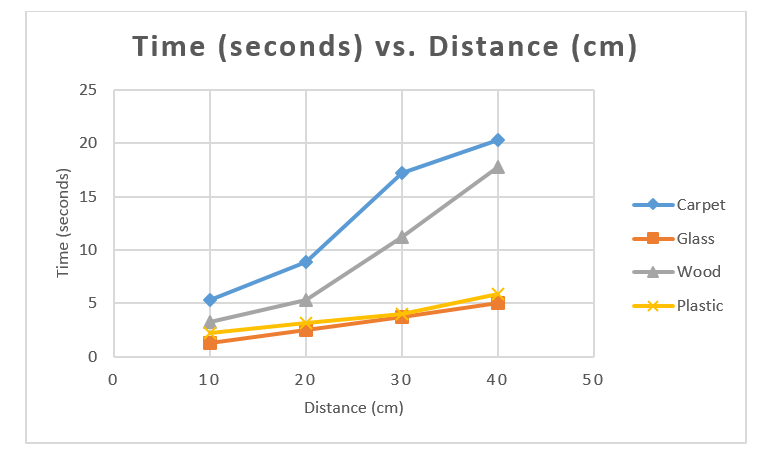What surface causes the least amount of friction?

Plastic

Wood

Carpet

Glass

Glass

Explanation:

Since the distance travelled, ramp angle and ball are all the same. The only thing that affects the time is the friction due to the surface of the ramp. The friction will cause the ball to move slower down the ramp and increase the time to travel the measured distance. Therefore the least amount of friction will cause the shortest travel time. The shortest travel time is on glass, which is the correct answer.

### Example Question #1236 : Act Science

Two scientists are concerned with producing electricity with magnets.

Scientist 1 believes that rotating a magnet within a coil of wires will produce electricity. Scientist 1 asserts that the larger the magnet the more electricity will be produced.

Scientist 2 believes that rotating the coil of wires around a magnet will produce electricity. Scientist 2 asserts that the more number of coils the more electricity will be produced.

The scientists conduct the following experiment. The scientists take four magnets, A, B, C and D; with A being the smallest magnet, B the next smallest, then C and the largest magnet was D. The scientist rotate the magnets at the same constant speed in the coils and record the number of milliamps produced. This was done in copper wiring with 20, 40 and 60 coils. When testing by rotating the coil around the magnet at the same speed of the magnet rotation produced the results recorded below.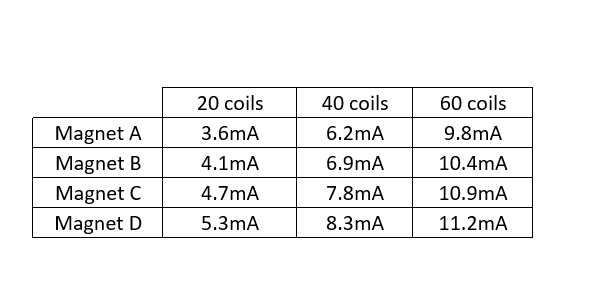How many more coils are needed around magnet A so that it will produce the same current as in experiments with magnet D?more coilsmore coilsmore coils

Cannot be determined from the given information

Cannot be determined from the given information

Explanation:

There is no information that would allow for one to determine the increase in the number of coils needed to create the same current output for the two magnets. Additionally, there is no relationship between current and number of coils that can be directly calculated. It can only be known qualitatively that the increase in coils causes an increase in the current output.

### Example Question #1237 : Act Science

A student is performing a science experiment for his class. The student creates a ramp that contains four different surfaces, carpet, glass, wood and plastic. The ramp is held at a constant angle and a ball is allowed to roll down the ramp. The student tests each material by releasing a ball at different distances from the bottom of the ramp and recording the time it takes for the ball to travel the distance of the ramp.If the ball released on the glass surface was to travel a distance of 50cm, about how long, in seconds, will it take for the ball to reach its destination?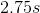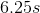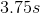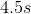Explanation:

One possible method of solving this problem is to extend the line that is given for the glass surface to 50cm. This will occur around 6.25 seconds. Another method of solving this is to notice that the longer the distance, the longer the time. This would eliminate 2.75, 3.75, and 4.5 seconds as they are shorter than the 5 second time at a distance of 40cm. This leaves teh correct answer of 6.25 seconds.

### Example Question #1238 : Act Science

Two scientists are concerned with producing electricity with magnets.

Scientist 1 believes that rotating a magnet within a coil of wires will produce electricity. Scientist 1 asserts that the larger the magnet the more electricity will be produced.

Scientist 2 believes that rotating the coil of wires around a magnet will produce electricity. Scientist 2 asserts that the more number of coils the more electricity will be produced.

The scientists conduct the following experiment. The scientists take four magnets, A, B, C and D; with A being the smallest magnet, B the next smallest, then C and the largest magnet was D. The scientist rotate the magnets at the same constant speed in the coils and record the number of milliamps produced. This was done in copper wiring with 20, 40 and 60 coils. When testing by rotating the coil around the magnet at the same speed of the magnet rotation produced the results recorded below.How can the highest current be produced as supported by the experiment?

Rotate both the magnet and the coils

Rotate both the magnet and the coils, while using the largest available magnet

Rotate both the magnet and the coils, while creating maximum number of coils

Create the maximum number of coils possible and use the largest magnet available

Create the maximum number of coils possible and use the largest magnet available

Explanation:

There is nothing mentioned about rotating both the magnet and the coils increasing the current. In the experiments, the larger the magnet the higher the current produced. Using the largest possible magnet will produce the highest possible current. In the experiment, the more coils the higher the current produced. So making the largest number of coils possible will produce the highest possible current. Using the largest magnet possible and the largest number of coils possible will produce the highest current.

### Example Question #1239 : Act Science

Metal wires are often used to conduct electricity. The electricity that flows through a wire is measured as "current." Therefore, a wire with an electric current is called a current carrying wire.

Current carrying wires also have a tendency to produce magnetic fields that circulate around them. The strength of that magnetic field is determined by the following equation whereis the magnetic field strength (Teslas),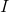is the electric current (Amps),is the distance (meters) of an object to the current carrying wire.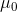is a constant.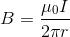Based on the given equation, what is the relationship between the strength of a magnetic field of a current carrying wire and that wire's electric current.

Inverse, exponential relationship

Inverse, linear relationship

Direct, exponential relationship

Direct, linear relationship

The correct answer is a direct, linear relationship. Although the equation may look complex, we just need to isolate the two variables specified-the magnetic field strength and the current. If we ignore all the other variables we get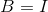meaning that the relationship is direct and since we are not working with exponents, it is linear.